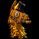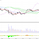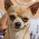# Functions Allowing Series As Length - PineCoders FAQ5849瀏覽
Pinescript requires many of its built-in functions to use a simple int as their period length, which entails the period length cannot vary during the script's execution. These functions allow using a series int or series float for their period length, which means it can vary on each bar.

The functions shared in this script include:
• Rolling sum: Sum( src ,p)
• Simple moving average: Sma( src ,p)
• Rolling variance: Variance( src ,p)
• Rolling standard deviation: Stdev( src ,p)
• Rolling covariance: Covariance(x,y,p)
• Rolling correlation: Correlation(x,y,p)

If p is a float then it is rounded to the nearest int.

How to Use the Script
Most of the functions in the script are dependent on the Sma function. The Correlation function uses the Covariance and Stdev functions. Be sure you include all the required functions in your script.

Make sure the series you use as the length argument is greater than 0, else the functions will return na. When using a series as length argument, the following error might appear:
`Pine cannot determine the referencing length of a series. Try using max_bars_back in the study or strategy function.`
This can be frequent if you use barssince(condition) where condition is a relatively rare event. You can fix it by including max_bars_back=5000 in your study declaration statement as follows:
`study("Title",overlay=true,max_bars_back=5000)`

Example
The chart shows the Sma, Stdev, Covariance and Correlation functions. The Sma uses the closing price as input and bars as period length where:

`bars = barssince(change(security(syminfo.tickerid,"D",close,lookahead=true)))`

The Stdev uses the closing price as input and bars + 9 as period length. The Covariance and Correlation use the closing price as x and bar_index as y, with bars + 9 as period length.

Tools and ideas for all Pine coders: http://www.pinecoders.com
Our Pine FAQ & Code: http://www.pinecoders.com/faq_and_code/

## 評論Great examples, thanks @PineCoders. And better late then never, best wishes and luck in 2020, cheers!Very elegant:
Sum(src,p) => a = cum(src), a - a****
-------------------------------------------------------------------
Sum(src,p) =>
a = cum(src) //This is the total accumulative
a - a*** //this the total a minus a from past to p periodThank you, this is really nice! Do you have any suggestion for the atr?BobAndrews
@BobAndrews, It's possible, I'll update the script soon with an Atr function.alexgrover
@alexgrover, the Atr-Function is beautiful, thanks again! 😍